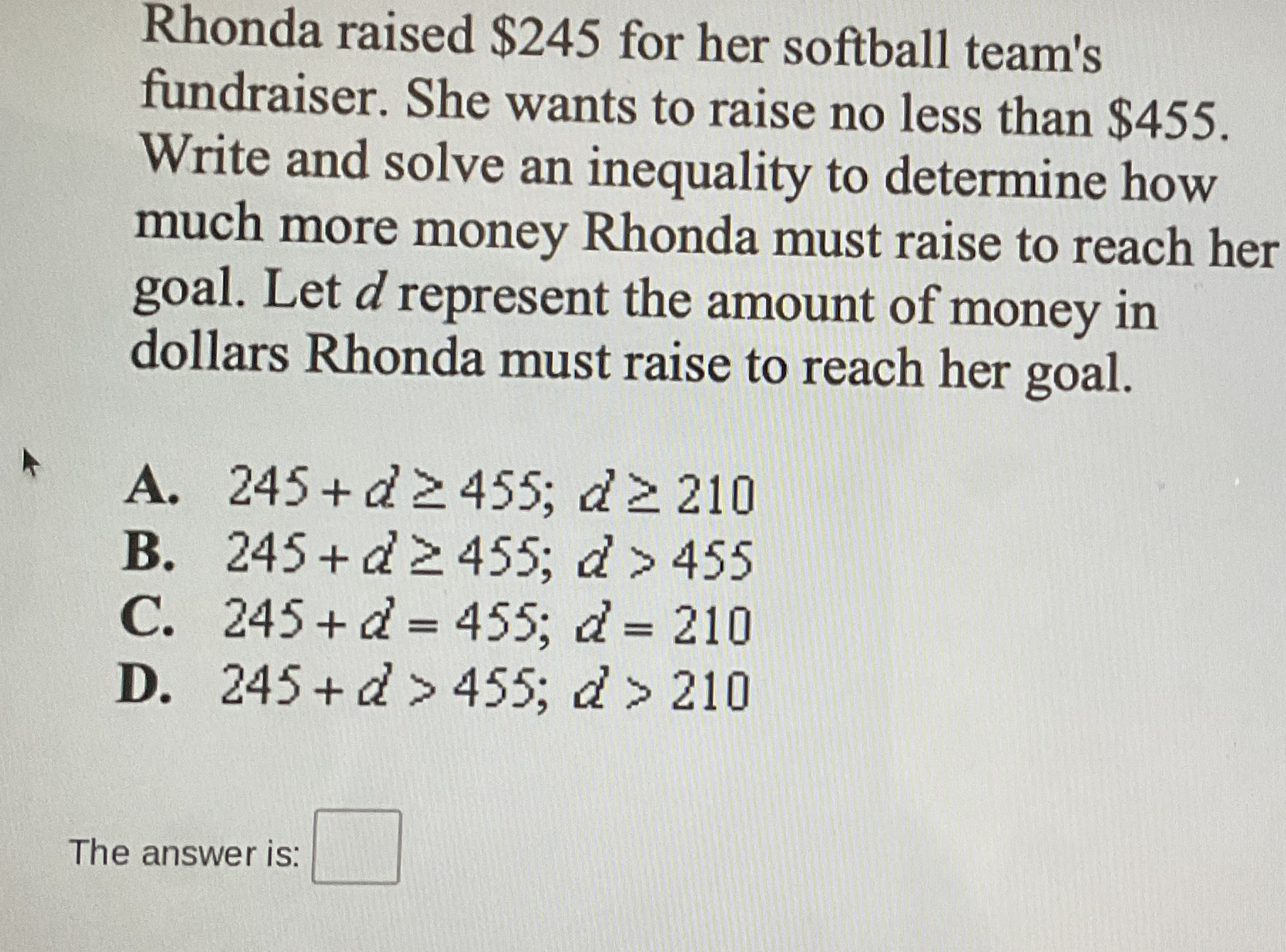### Still have math questions?

Algebra
QuestionRhonda raised $$\ 245$$ for her softball team's fundraiser. She wants to raise no less than $$\ 455$$ . Write and solve an inequality to determine how much more money Rhonda must raise to reach her goal. Let $$d$$ represent the amount of money in dollars Rhonda must raise to reach her goal. A. $$245 + d \geq 455 ; d \geq 210$$

B. $$245 + d \geq 455 ; d > 455$$

C. $$245 + d = 455 ; d = 210$$

D. $$245 + d > 455 ; d > 210$$